## Standardized tests: In a particular year, the mean score on the ACT test was 22.5 and the standard deviation was 5.3. The mean score on the

Question

Standardized tests: In a particular year, the mean score on the ACT test was 22.5 and the standard deviation was 5.3. The mean score on the SAT mathematics test was 526 and the standard deviation was 101. The distributions of both scores were approximately bell-shaped. Round the answers to at least two decimal places. Part: 0 / 40 of 4 Parts Complete Part 1 of 4 Core for an ACT score of 16. The z-score for an ACT score of 16 is Jose’s ACT score had a -score of . What was his ACT score?

in progress 0
1 month 2021-08-04T19:28:39+00:00 1 Answers 2 views 0

The z-score for an ACT score of 16 is -1.23.

His ACT score was of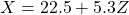, considering that Z is his z-score.

Step-by-step explanation:

Z-score:

In a set with mean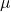and standard deviation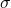, the z-score of a measure X is given by: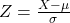The Z-score measures how many standard deviations the measure is from the mean. After finding the Z-score, we look at the z-score table and find the p-value associated with this z-score. This p-value is the probability that the value of the measure is smaller than X, that is, the percentile of X. Subtracting 1 by the p-value, we get the probability that the value of the measure is greater than X.

ACT:

Mean of 22.5, standard deviation of 5.3, so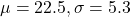The z-score for an ACT score of 16 is

Z when x = 16. So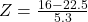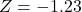The z-score for an ACT score of 16 is -1.23.

Jose’s ACT score had a Z-score of Z. What was his ACT score?

This is X, considering Z his z-score. So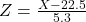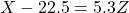His ACT score was of, considering that Z is his z-score.